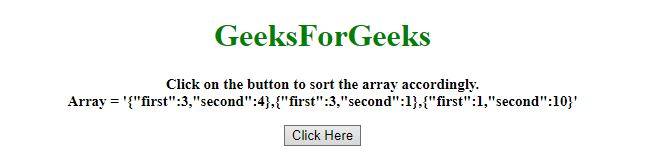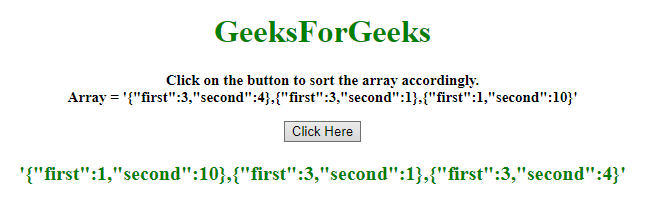# How to sort an array of object by two fields in JavaScript ?

• Last Updated : 13 Dec, 2019

Given an array of objects and the task is to sort the array elements by 2 fields of the object. There are two methods to solve this problem which are discussed below:

Approach 1:

Hey geek! The constant emerging technologies in the world of web development always keeps the excitement for this subject through the roof. But before you tackle the big projects, we suggest you start by learning the basics. Kickstart your web development journey by learning JS concepts with our JavaScript Course. Now at it's lowest price ever!

• First compare the first property, if both are unequal then sort accordingly.
• If they are equal then do the same for the second property.

Example: This example implements the above approach.

 ` ``<``html``> `` ` `<``head``> ``    ``<``title``> ``        ``How to sort an array of object``        ``by two fields in JavaScript ?``    ```` `` ` `<``body` `style` `= ``"text-align:center;"``> ``     ` `    ``<``h1` `style` `= ``"color:green;"` `> ``        ``GeeksForGeeks ``    ````     ` `    ``<``p` `id` `= ``"GFG_UP"` `style = "font-size:``        ``15px; font-weight: bold;">``    ````     ` `    ``<``button` `onclick` `= ``"gfg_Run()"``> ``        ``Click Here``    ````     ` `    ``<``p` `id` `= ``"GFG_DOWN"` `style = "color:green;``        ``font-size: 20px; font-weight: bold;">``    ````     ` `    ``<``script``>``        ``var el_up = document.getElementById("GFG_UP");``        ``var el_down = document.getElementById("GFG_DOWN");``        ``var arr = [``            ``{first: 3, second: 4},``            ``{first: 3, second: 1},``            ``{first: 1, second: 10}``        ``];``         ` `        ``el_up.innerHTML = "Click on the button to sort "``                ``+ "the array accordingly.<``br``>Array = '"``                ``+ JSON.stringify(arr) + ", "``                ``+ JSON.stringify(arr) +", "``                ``+ JSON.stringify(arr) + "'";``         ` `        ``arr.sort(function (a, b) {``            ``var af = a.first;``            ``var bf = b.first;``            ``var as = a.second;``            ``var bs = b.second;``             ` `            ``if(af == bf) {``                ``return (as < ``bs``) ? -1 : (as > bs) ? 1 : 0;``            ``} else {``                ``return (af < ``bf``) ? -1 : 1;``            ``}``        ``});``         ` `        ``function gfg_Run() {``            ``el_down.innerHTML` `= ``"'"` `+ JSON.stringify(arr)``                    ``+ ", " + JSON.stringify(arr) + ", "``                    ``+ JSON.stringify(arr) + "'";``        ``} ``    `` `` `` ` ``

Output:

• Before clicking on the button:• After clicking on the button:Approach 2:

• First compare the first property, If both are unequal then sort accordingly.
• If they are equal then do the same for the second property, this example is following the same approach but using OR Gate to reduce the code.

Example: This example implements the above approach.

 ` ``<``html``> `` ` `<``head``> ``    ``<``title``> ``        ``How to sort an array of object``        ``by two fields in JavaScript ?``    ```` `` ` `<``body` `style` `= ``"text-align:center;"``>``     ` `    ``<``h1` `style` `= ``"color:green;"` `> ``        ``GeeksForGeeks ``    ````     ` `    ``<``p` `id` `= ``"GFG_UP"` `style` `=``        ``"font-size: 15px; font-weight: bold;"``>``    ````     ` `    ``<``button` `onclick` `= ``"gfg_Run()"``> ``        ``Click Here``    ````     ` `    ``<``p` `id` `= ``"GFG_DOWN"` `style = "color:green;``        ``font-size: 20px; font-weight: bold;">``    ````     ` `    ``<``script``>``        ``var el_up = document.getElementById("GFG_UP");``        ``var el_down = document.getElementById("GFG_DOWN");``         ` `        ``var arr = [``            ``{first: 3, second: 4},``            ``{first: 3, second: 1},``            ``{first: 1, second: 10}``        ``];``         ` `        ``el_up.innerHTML = "Click on the button to sort "``                ``+ "the array accordingly.<``br``>Array = '"``                ``+ JSON.stringify(arr) + ", " + ``                ``JSON.stringify(arr) +", " +``                ``JSON.stringify(arr) + "'";``         ` `        ``arr.sort(function (a, b) { ``            ``return a.first - b.first || a.second - b.second;``        ``});``         ` `        ``function gfg_Run() {``            ``el_down.innerHTML = "'" + JSON.stringify(arr)``                    ``+ ", " + JSON.stringify(arr) + ", "``                    ``+ JSON.stringify(arr) + "'";``        ``} ``    `` `` `` ` ``

Output:

• Before clicking on the button:• After clicking on the button:My Personal Notes arrow_drop_up